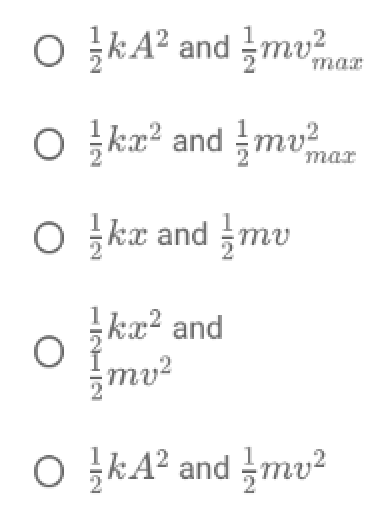# Problem: Which of the following are proper expressions for the total energy of an object in SHM?

###### FREE Expert Solution

Total energy in SHM:

$\overline{){\mathbf{E}}{\mathbf{=}}\frac{\mathbf{1}}{\mathbf{2}}{\mathbf{k}}{{\mathbf{A}}}^{{\mathbf{2}}}{\mathbf{=}}\frac{\mathbf{1}}{\mathbf{2}}{\mathbf{m}}{{{\mathbf{v}}}_{\mathbf{m}\mathbf{a}\mathbf{x}}}^{{\mathbf{2}}}}$

79% (374 ratings)###### Problem Details

Which of the following are proper expressions for the total energy of an object in SHM?